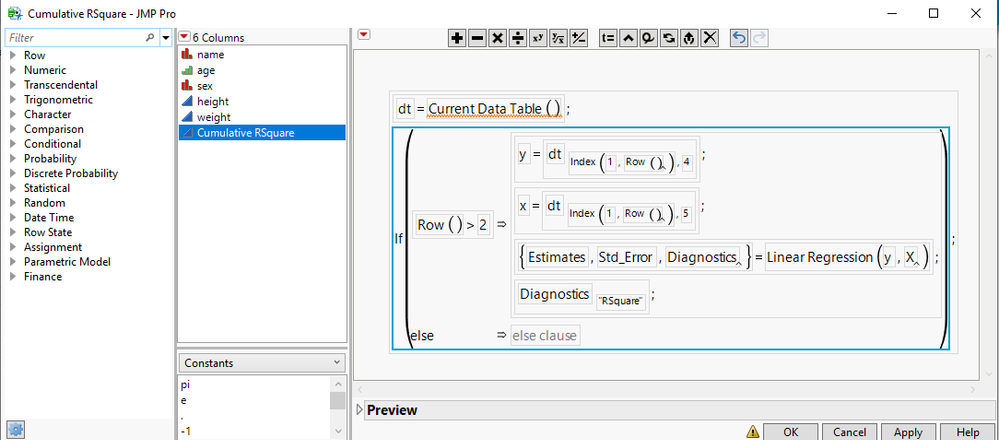Our World Statistics Day conversations have been a great reminder of how much statistics can inform our lives. Do you have an example of how statistics has made a difference in your life? Share your story with the Community!
Choose Language Hide Translation Bar
Highlighted

## Re: Calculating linear regression slope in formula

Hi,

Is there any plan to put slope and intercept into formula builder of a future JMP version w/ a more straight forward direct function ?

thx.

Highlighted

## Re: Calculating linear regression slope in formula

Take a look at the Linear Regression() function, which could be used in a formula.  It might be something you can use.

Jim
Highlighted

## Re: Calculating linear regression slope in formula

hi ...

You mentioned "Linear Regression() function, which could be used in a formula" ...and getting a slope from a formula fitting to provided data set being the final objective, where would one go find Linear Regression() function or any help ?

Do you have any example ?

thanks

Highlighted

## Re: Calculating linear regression slope in formula

Select Help > Scripting Index. Enter "regression" in the filter box. You should find the Linear Regression() function in the second list. Select it to see information about this function on the right.

Learn it once, use it forever!
Highlighted

## Re: Calculating linear regression slope in formula

I am not looking for a script.
I am looking for a formula application (i.e. using formula builder)
Highlighted

## Re: Calculating linear regression slope in formula

A JMP formula can pretty much be anything you want it to be.  My pointing you to the Linear Regression() function in the Scripting Index, happens to show it's example in the form of a script, but the function can also be used in a formula.  Below is a silly example, of calculating the regression for first rows 1-3, then 1-4, 1-5, etc. for all of the rows in the Big Class data table.  Here is the formula...….attached in the data table with the new formula column addedJim
HighlightedJeff_PerkinsonCommunity Manager

## Re: Calculating linear regression slope in formula

Can you give us some more background on what you're looking for?

Formulas, generally speaking, work across rows of a data table, evaluating one per row with column values from that row as inputs.

So, by asking for a regression function in the formula editor, one would expect that you had a matrix of input values in each row of your data table. Perhaps that's what you've got but that's an unusual structure.

So, with some more information we may be able to help you understand how you could use a formula for this, or perhaps, point you in a direction outside of the formula editor.

-Jeff
Highlighted

## Re: Calculating linear regression slope in formula

Hello JMP team ...

Nelson's approach is very likely what I was looking for. I am conversing w/ him on a similar need with mann kendall tau test.

My input is not in a single row.

My data is typically contains several parameters each measured at a different time step. Tidy data format; parameters are in columns, each row is a different time state - i.e. chronology is in rows.

I am looking into many statistical functions measuring trend in one of these or a fit versus some other X in time but as a function of rolling windows. So instead of regressing the whole history of X vs Y for instance, I like a logic that looks into regression of the two within constant past N time steps. Each row then re-evaluates and gets me a new result. These could a slope from linear regression, or a tau test from a non-parametric MK test or some other stat output.

Many times, we do not know when the correlation between the X's have changed, so this is one attempt to look into the impact of time for any analysis at hand. N mentioned above can be changed to various values to examine short term vs mid/long terms trends.

I am ok w/ scripts, they are not my preference unless the computations can great benefit a method offered only by scripts.

My preference is generally to put the calculations into formulaes and have them maintained within the data frames.

Until today, did not know that script structures could be deployed within formulaes.

That probably was the main cause of my confusion.

Highlighted

## Re: Calculating linear regression slope in formula

You said, "Many times, we do not know when the correlation between the X's have changed, so this is one attempt to look into the impact of time for any analysis at hand." Have you tried the Functional Data Explorer in JMP Pro? It might be useful for detection a change in the shape through the functional principal components.

Learn it once, use it forever!
Highlighted

## Re: Calculating linear regression slope in formula

I know.

My point is that the best way to start learning about functions that you might use in a formula is with the Scripting Index.

Learn it once, use it forever!
Article Labels

There are no labels assigned to this post.